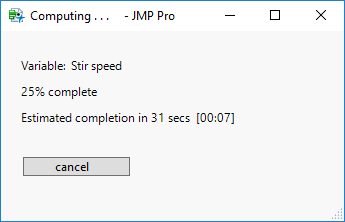Our World Statistics Day conversations have been a great reminder of how much statistics can inform our lives. Do you have an example of how statistics has made a difference in your life? Share your story with the Community!
Choose Language Hide Translation Bar
Highlighted

## Fastest way to edit lists of strings

I'm trying to filter a list of lists of strings.  As an example:

I've got 2 lists, for filter 1, I'd like to concatenate the two lists iff the 2nd list doesn't match the first lists characters after the third.  So for instance "a3232", "32" would violate this, but "a32323", "32" would not.

for filter 2 I'd like to remove hyphens (or whatever character fits your fancy).

I've made a quick example function that returns the filtered list but in real code this takes quite some time.

Wondering if anyone has had any luck doing similar things faster.

``````Names Default to here(1);
x = {{"a2232", "b12", "c-", "d3341234", "e12647859"}, {"32", "32", "54", "", "64"}};

filterfunc = function({bothlists},
{DEFAULT LOCAL},
f = Expr(column(dt, "1")[]||column(dt, "2")[]);

dt = New Table("Test", private,
New Column("1", character, set values(bothlists)),
New Column("2", character, set values(bothlists)),
//New Column("First 3", character, Formula(Left(column(dt, "1")[], 3))),
New Column("Remainder", character, Formula(Right(column(dt, "1")[], length(column(dt, "1")[])-3))),
New Column("Filter", character ),
);

filter1 = 1; 	// filter to remove duplicates from of remainder
//after the first 3 of column 1 and the total of column 2
filter2 = 1; //filter to remove hyphens
if(filter1,
Substitute into(f,
Expr(column(dt, "2")[]),
Expr(if(Column(dt, "Remainder")[] != column(dt, "2")[],
column(dt, "2")[],
""
))
)
);

if(filter2,
Substitute into(f,
nameexpr(f),
EvalExpr(Substitute(Expr(nameexpr(f)), "-", ""))
)
);

Column(dt, "Filter") << Formula(nameexpr(f));
dt << Run Formulas;
values = Column(dt, "Filter") << Get Values;
close(dt, no save);
values;
);

st = HPTime(); //start of routine
filtered_stuff = filterfunc(x);
tot = HPTime() - st;
show(tot);``````

I've thought about premaking the filter selections but as the number of filters add up this becomes a 2^n problem I think and I'm worried about start up time.

Vince Faller - Predictum
5 REPLIES 5
HighlightedDavid_Burnham
Super User

## Re: Fastest way to edit lists of strings

I think the over-abundance of evals etc have made this code difficult for me to understand so I've created a simplified version.

Here is the simplified code, just focusing on the 2nd filter, using your coding structure:

``````t1 = HpTime();
bothlists = {{"a2232", "b12", "c-", "d3341234", "e12647859"}, {"32", "32", "54", "", "64"}};
dt = New Table("Test",Private,
New Column("1", character, Set Values(bothlists)),
New Column("2", character, Set Values(bothlists)),
New Column("Filter", character)
);
f = Expr(column(dt, "1")[]||column(dt, "2")[]);
Substitute into(f,
nameexpr(f),
EvalExpr(Substitute(Expr(nameexpr(f)), "-", ""))
);
Column(dt, "Filter") << Formula(nameexpr(f));
dt << Run Formulas;
values = Column(dt, "Filter") << Get Values;
t2 = HpTime();
show(t2-t1);
show(values);``````

On my computer the HP Time Difference is about 2000.

Next, I rewrote the code to avoid the use of expressions and evals etc:

``````t1 = HpTime();
bothlists = {{"a2232", "b12", "c-", "d3341234", "e12647859"}, {"32", "32", "54", "", "64"}};
dt = New Table("Test", Private,
New Column("1", character, Set Values(bothlists)),
New Column("2", character, Set Values(bothlists)),
New Column("Filter", character)
);

Column(dt, "Filter") << Formula(
Substitute( :Name( "1" ) || :Name( "2" ), "-", "" )
);
dt << Run Formulas;
values = Column(dt, "Filter") << Get Values;
t2 = HpTime();
show(t2-t1);
show(values);``````

This has about a 25% performance improvement with the reported HP TIme Difference being ~1500.

Tables are expensive things to use, even if private, so here is some code that doesn't use them:

``````t1 = HpTime();
bothlists = {{"a2232", "b12", "c-", "d3341234", "e12647859"}, {"32", "32", "54", "", "64"}};
lst = {};
For (i=1,i<=NItems(bothlists),i++,
lst[i] = Substitute( bothlists[i] || bothlists[i], "-", "" )
);

t2 = HpTime();
show(t2-t1);
show(lst);``````

The reported HP Time Difference for this code is less than 100.

-Dave
Highlighted

## Re: Fastest way to edit lists of strings

Which version of JMP are you using?  I know lists got enhanced in 13 but I've got a list of 1,000,000 items so doing them in a for loop was really slow (I'm running 12.2).

Also, I've got to do all the filters simultaneously so I have to build my filter expression first dynamically then I have to evaluate it.

Vince Faller - Predictum
HighlightedDavid_Burnham
Super User

## Re: Fastest way to edit lists of strings

I increased the number of items in the lists to 1 million.  The first method took 14 seconds, the second method 7 seconds and the third method 5 seconds.  This is with version 13, so it looks like they've done a good job of improving the performance of large lists.

-Dave
HighlightedDavid_Burnham
Super User

## Re: Fastest way to edit lists of strings

One plus point of iterating over items in a list is that you can construct a dialog window showing progress:Slow calculations are much more acceptable to users when they know how long they have to wait, and when they have an option to abort if the completion time is longer than they anticipated.

-Dave
Highlighted

## Re: Fastest way to edit lists of strings

This isn't a calculation really, it's more of a gui element so speed needs to be fast.  Here are my results when I tried it.

Running it with a loop in JMP 13.1: 7.7 seconds

Running with the data table in JMP 13 3.3 seconds

Running with loop in JMP 12.2:  2+ minutes

running with data table in JMP 12.2:  3.5 seconds

I don't know how you got such a speed increase with the loop.

Vince Faller - Predictum
Article Labels

There are no labels assigned to this post.